# Theodorsen integral equation

Theodorsen's integral equation [a7] is a well-known tool for computing numerically the conformal mappingof the unit disconto a star-like regiongiven by the polar coordinates,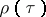of its boundary. The mappingis assumed to be normalized by,. It is uniquely determined by its boundary correspondence function, which is implicitly defined by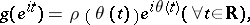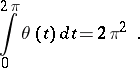Theodorsen's equation follows from the fact that the function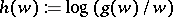is analytic inand can be extended to a homeomorphism of the closure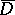onto the closure. It simply states that the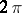-periodic function:is the conjugate periodic function of: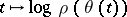, that is,, whereis the conjugation operator defined on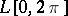by the principal value integral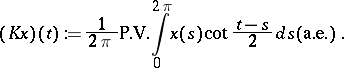When restricted to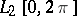,is a skew-symmetric endomorphism of normwith a very simple diagonal representation in Fourier space: whenhas the real Fourier coefficients,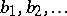, thenhas the coefficients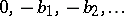,.

Hence, while Theodorsen's integral equation is normally written as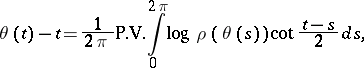for practical purposes the conjugation is executed by transformation to Fourier space:is approximated by a trigonometric polynomial of degree, whose Fourier coefficients are quickly found by the fast Fourier transform, which then can also be applied to determine values at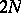equi-spaced points of the trigonometric polynomial that approximates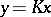(cf. also Fourier series). Before the fast Fourier transform became the standard tool for this discrete conjugation process, the transition from the values ofto those ofwas based on multiplication by a matrix, called the Wittich matrix in [a1]. The fast Fourier transform meant a cost reduction fromtooperations per iteration.

Until the end of the 1970s the recommendation was to solve a so-obtained discrete version of Theodorsen's equation by fixed-point (Picard) iteration, an approach that is limited to Jordan regions with piecewise differentiable boundary satisfying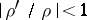, and is very slow when the boundis nearly attained. Other regions, like those from airfoil design, which was the standard application targeted by T. Theodorsen, could be handled by using first a suitable preliminary conformal mapping, which turned the exterior of the wing cross-section into the exterior of a Jordan curve that is close to a circle; see [a6], Chapt. 10. Moreover, for this application, the equation has to be modified slightly to map the exterior of the disc onto the exterior of a Jordan curve.

M. Gutknecht [a4], [a2] extended the applicability of Theodorsen's equation by applying more refined iterative methods and discretizations, and O. Hübner [a5] improved the convergence order from linear to quadratic by adapting R. Wegmann's treatment of a similar equation obtained by choosinginstead. Wegmann's method [a9], [a10] applies the Newton method and solves the linear equation for the corrections by interpreting it as a Riemann–Hilbert problem that can be solved with four fast Fourier transforms.

A common framework for conformal mapping methods based on function conjugation is given in [a3]; Theodorsen's restriction to regions given in polar coordinates can be lifted. Both Theodorsen's [a8] and Wegmann's [a11] equations and methods can be extended to the doubly connected case.